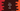# delete vs free in C++ with examples## delete vs free in C++:

In this post, we will learn the main differences between free and delete in C++. delete is an operator but free is a function. Also, both seem similar but they are different in many ways. Let’s learn the differences between free and delete.

### What is delete operator:

The delete operator is mostly used in C++. This operator is used to dynamically de-allocate the memory. This operator is used for pointers that are pointing to memory locations allocated using new or if this is a NULL pointer.

If we use delete, it calls the destructor before the memory is released. Note that it will not destroy the pointer, it will only de-allocate the memory pointed by the pointer. We can use it with arrays and other objects which were created or memory was allocated by the new keyword.

#### Syntax of delete:

Below is the syntax of the delete operator:

``delete pointer``

or

``delete []pointer``

The first one is used to de-allocate the memory for a single object and the second one is used for arrays.

#### Example program:

Let’s understand how delete works with an example program:

``````#include <iostream>
#include <string>
using namespace std;

int main()
{
string *str;
str = new string;

*str = "Hello World";

cout<<*str<<endl;

delete str;

cout<<*str<<endl;

return 0;
}``````

In this example, we created a string pointer str and assigned a string to this pointer. The value of str is printed before and after we are using delete.

If you run this program, it will print:

``````Hello World
@�y%���d``````

As you can see, the content of str is removed.

### free function:

The free function is defined in the stdlib.h header file. This function can be used to de-allocate memory dynamically. This function can be used to delete the memory if it was allocated by malloc function or NULL pointer.

The syntax of free is defined as like below:

``free(p)``

Where p is a pointer pointing to the memory block.

#### Example program:

Let’s try the free function with an example program:

``````#include <iostream>
#include <cstdlib>
using namespace std;

int main()
{
int *i;
i = (int*)malloc(1*sizeof(int));

*i = 10;

cout<<*i<<endl;

free(i);

cout<<*i<<endl;

return 0;
}``````

In this program,

• We created an integer pointer i. This is allocated by using malloc.
• We assigned 10 to i.
• The value of i is printed before and after free is called.

If you run this program, it will print output as like below:

``````10
-908738512``````

So, after we called free, the memory is deleted and it printed a garbage value.

### Differences between delete and free:

Following are the differences between delete and free:

delete free
delete is an operator free is a library function
delete operator dynamically de-allocates the memory free function destroys the memory at runtime
delete operator can delete a pointer which is allocated using new or NULL pointer free function can delete a pointer which is allocated using malloc, calloc, realloc or NULL pointer
delete operator calls the destructor of the class after it de-allocates the memory free function only de-allocates the memory without calling the destructor
delete is faster free is slower than delete